# A uniform thin rod of length 0.65 m and mass 3.4 kg can rotate in a horizontal plane about a vert...

A uniform thin rod of length 0.65 m and mass 3.4 kg can rotate in a horizontal plane about a vertical axis through its center. The rod is at rest when a 3.8 g bullet traveling in the rotation plane is fired into one end of the rod. As viewed from above, the bullet's path makes angle θ = 60° with the rod. If the bullet lodges in the rod and the angular velocity of the rod is 11.0 rad/s immediately after the collision, what is the bullet's speed just before impact?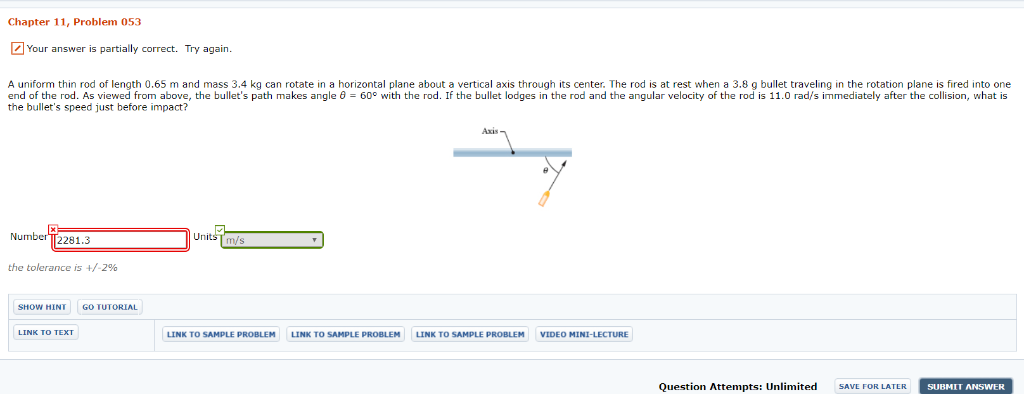Chapter 11, Problem 053 Your answer is partially correct. Try again. A uniform thin rod of length 0.65 m and mass 3.4 kg can rotate in a horizontal plane about a vertical axis through its center. The rod is at rest when a 3.8 g bullet traveling in the rotation plane is fired into one end of the rod. As viewed from above, the bullet's path makes angle θ 60° with the rod. If the bullet lodges in the rod and the angular velocity of the rod is 11.0 rad/s immediately after the collision, what is the bullet's speed just before impact? Number T2281.3 the tolerance is +/-296 SHOW HINT GO TUTORIAL LINK TO SAMPLE PROBLEM LINK TO SAMPLE PROBLEMLINK TO SAMPLE PROBLEM VIDEO MINI-LECTURE Question Attempts: Unlimited SAVE FOR LATER SUBMIT ANSWER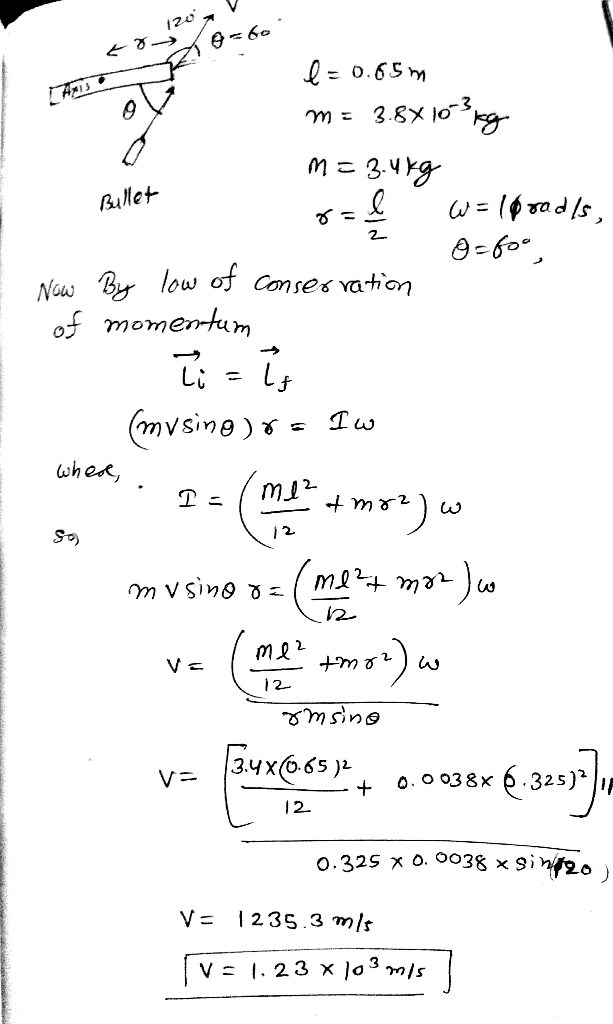##### Add Answer of: A uniform thin rod of length 0.65 m and mass 3.4 kg can rotate in a horizontal plane about a vert...
Similar Homework Help Questions
• ### Chapter 11, Problem 053 GO A uniform thin rod of length 0.61 m and mass 3.3...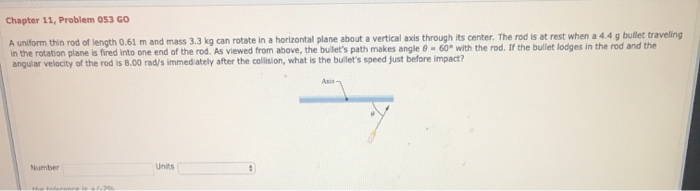Chapter 11, Problem 053 GO A uniform thin rod of length 0.61 m and mass 3.3 kg can rotate in a horizontal plane about a vertical axis through its center. The rod is at rest when a 4.4 g bullet traveling in the rotation plane is fired into one end of the rod. As viewed from above, the bullet's path makes angle 9 -60° with the rod. If the bullet lodges in the rod and the angular velocity of the...

• ### HW 8 chapt 11

A uniform thin rod of length 0.60 m and mass 3.0kg can rotate in a horizontal plane about a vertical axis throughits center. The rod is at rest when a 3.0g bullet traveling in therotation plane is fired into one end of the rod. As viewed fromabove, the bullet's path makes angle θ = 60° withthe rod. If thebullet lodges in the rod and the angular velocityof the rod is 7 rad/s immediatelyafter the collision, what is the bullet's speed...

• ### Bullet hits a uniform thin rod

A uniform thin rod of length 0.60 m and mass 2.5kg can rotate in a horizontal plane about a vertical axisthrough its center. The rod is a rest when a3.0-g bullet travelingin the horizontal plane of the rod is fired into one end of therod. The direction of the bullet velocity makes an angle of 60°with the rod. Ifthe bullet lodges in the rod and the angularvelocity of the rod is 9.0 rad/s immediately after thecollision, what is the magnitude...

• ### bullet hits a uniform thin rodA uniform thinrod of length 0.75 m and mass 3.5 kg can rotate in a horizontal plane about a vertical axis through its center. The rod is a rest when a 3.0-g bullet traveling inthe horizontal plane of the rod is fired into one end of the rod. As viewed from above, the direction of the bullet velocity makes an angle of 60° with the rod(see the figure). If the bullet lodges in the rod and the angular velocity of...

• ### Bullet hitting a uniform thin rodA uniform thinrod of length 0.40 m and mass 6.0 kg can rotate in a horizontal plane about a vertical axis through its center. The rod is a rest when a 3.0-g bullet traveling inthe horizontal plane of the rod is fired into one end of the rod. As viewed from above, the direction of the bullet velocity makes an angle of 60° with the rod(see the figure). If the bullet lodges in the rod and the angular velocity of...

• ### Need HELP on this one, thanks in advance!! A uniform thin rodA uniform thin rod of length 0.45 m and mass 4.0kg can rotate in a horizontal plane about a vertical axisthrough its center. The rod is a restwhen a 3.0-g bullet travelingin the horizontal plane of the rod is fired into one end of therod. As viewed from above, the direction of the bullet velocitymakes anangle of 60° with the rod (see the figure). If thebullet lodges in the rod and the angular velocity of the rod is 8.0rad/s immediately...

• ### Stuck!! Will Reward YOU WELL!! bullet hits A uniform thin rodA uniform thin rod of length 0.45 m and mass 4.0kg can rotate in a horizontal plane about a vertical axisthrough its center. The rod is a restwhen a 3.0-g bullet travelingin the horizontal plane of the rod is fired into one end of therod. As viewed from above, the direction of the bullet velocitymakes anangle of 60° with the rod (see the figure). If thebullet lodges in the rod and the angular velocity of the rod is 8.0rad/s immediately...

• ### Rotation of Rigid Bodies & Angular Momentum 11

A uniform thin rod of length 0.50 m and mass 4.0 kg can rotatein a horizontal plane about a vertical axis through its center. Therod is at rest when a 3.0 g bullet traveling in the rotation plane isfired into one end of the rod. As viewed from above, the bullet’spath makes angle = 60degrees with the rod (SeeFigure). If the bullet lodges inthe rod and the angular velocityof the rod is 10 rad/simmediately after the collision,what is the bullet’s...

• ### Q21 (15 points): A uniform rod of mass m 1.5 kg and length d- 2.0 m...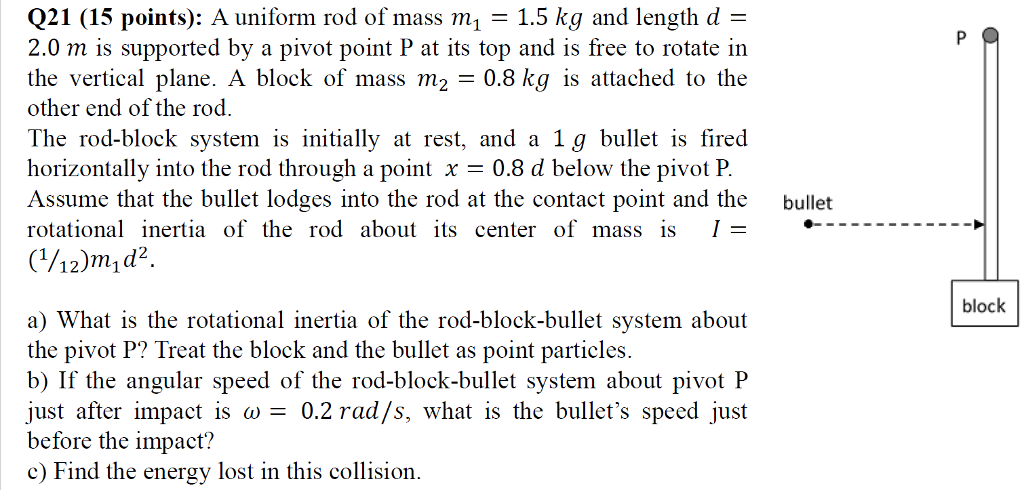Q21 (15 points): A uniform rod of mass m 1.5 kg and length d- 2.0 m is supported by a pivot point P at its top and is free to rotate iın the vertical plane. A block of mass m2 0.8 kg is attached to the other end of the rod. The rod-block system is initially at rest, and a 1g bullet is fired horizontally into the rod through a point x 08 d below the pivot P Assume that...

• ### Part A is completed (0.205). I need parts B and C (circled in yellow). Incorrect answers which I tried are circled in red (6%) Problem 16: A rod of niass M-3.05 krt and length L can rotate about a...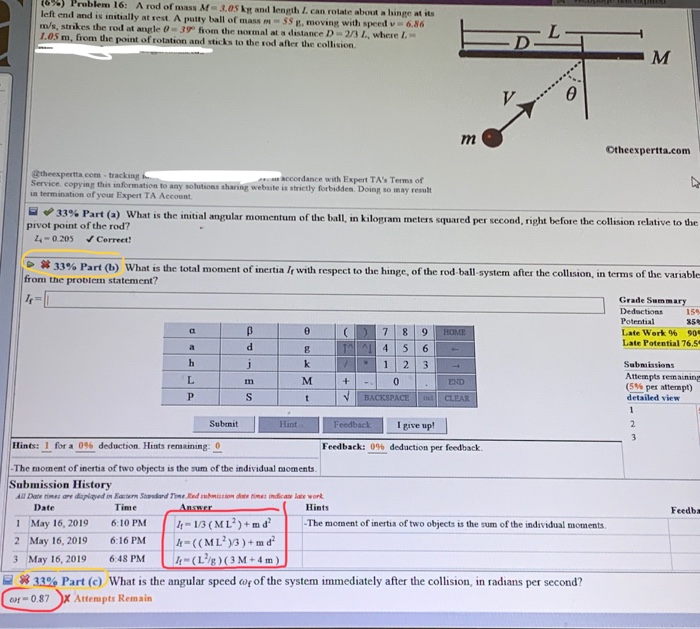Part A is completed (0.205). I need parts B and C (circled in yellow). Incorrect answers which I tried are circled in red (6%) Problem 16: A rod of niass M-3.05 krt and length L can rotate about a lunge at sts left end and is initially at rest. A putty ball of mass m5s g, moving with speed v 6.86 m/s, strikes the rod at angle 0- 39 from the normal at a distance D 23 L, where L...

Need Online Homework Help?